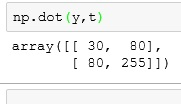# Python: Numpy

First off, CONGRATS for making it this far. Numpy really signifies the first step in real data science with Python.

Numpy is a library that adds advanced mathematical capabilities to Python. If you are using the Anaconda release of iPython, you already have numpy installed. If not, you will need to go over to their site and download it. Link to numpy site: www.numpy.org

Numpy adds multi-dimensional array capabilities to Python. It also provides mathematical tools for dealing with these arrays.

## Array

What is an array? In different programming languages, arrays are equivalent to lists in Python. In fact, a single dimension array in Python can be used interchangeably with lists in most cases.

What makes arrays special in Python is the ability to make multidimensional arrays. Those who have taken math courses like Linear Algebra will better know multidimensional arrays by the term matrix. And if you paid attention in Linear Algebra, you will know there is a lot of cool things you can do with matrices.

For those who didn’t take Linear Algebra (you probably went out and made friends and had a social life), don’t worry, I will fill you in on what you need to know.

## Numpy

Let’s start by creating a single dimension array

• import numpy as np   — imports numpy into your notebook, we set the alias to np
• x = np.array([8,7,4])  — array is a method of np, so the syntax is np.array. **note the [] inside the ()
• x = you call array just like lists
• x.shape = shows you shape of your array, since we are single dimension, the answer is a single number – **note 3L – the L indicates integer in PythonNow let’s make a 2 x 3 matrix. When describing a matrix (or multi-dim array) the first number indicates the number of elements across and the second number indicates down. This is standard mathematical notation.

You can see I create a new row by enclosing the number sets in [] separated by a ‘,’

Now when want to call an item from the multi-dim array, you need to use 2 index numbers. y[0,1] = y[row, column]## np.arange()

np.arange() is a command we can use to auto populate an array.

1. np.arang(5) –create a one – dim array with 5 elements
2. np.arange(10).reshape(2,5) – reshape lets you convert a one-dim array into a multi-dim array## Transpose

Transposing a matrix means flipping it on its axis. This comes in handy in applications like multi-variate regressions. To transpose our array in Python, we use the “.T” method## dot Product

Performing a dot product on a matrix is a useful calculation, but it is very time consuming. Numpy makes it easy though.If you need a brush up on dot products, this is a great link: matrix multiplication

If you enjoyed this lesson, click LIKE below, or even better, leave me a COMMENT.

Last Lesson: Create. Import, and use Modules

Next Lesson: Numpy Part II